# The coefficients of friction between the block and the rail are μN = 0.30 and μk = 0.25. Knowing that θ =65° determine the smallest value of P required (a) to start the block up the rail, (b) to keep it from moving down.

Question-AnswerCategory: Engineering MechanicsThe coefficients of friction between the block and the rail are μN = 0.30 and μk = 0.25. Knowing that θ =65° determine the smallest value of P required (a) to start the block up the rail, (b) to keep it from moving down.

The coefficients of friction between the block and the rail are μN = 0.30 and μk = 0.25. Knowing that θ =65° determine the smallest value of P required (a) to start the block up the rail, (b) to keep it from moving down.Step: 1

(a)
Draw the free body diagram of the block.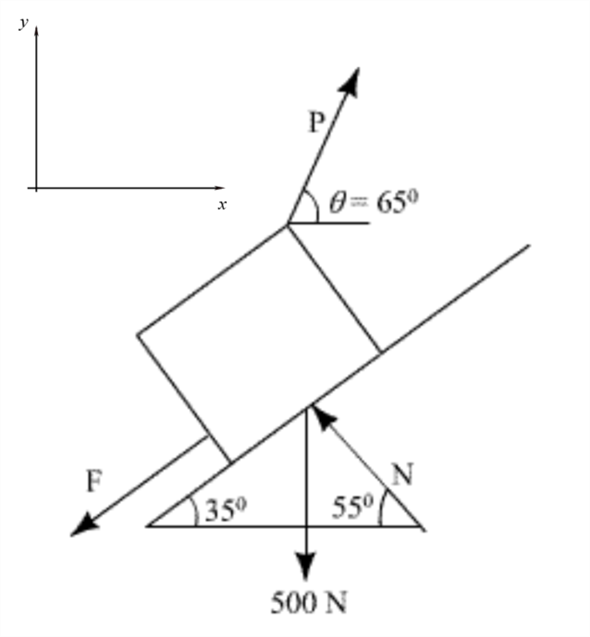Step: 2

Assume that the force in the positive x-direction is positive.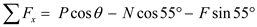Apply the equilibrium condition,…… (1)
Assume that the force in the positive y-direction is positive.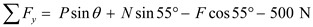Apply the equilibrium condition,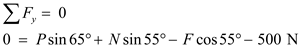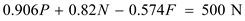…… (2)

Step: 3

The maximum value of frictional force,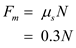As the block is starting to move up the rail (impending motion), frictional force: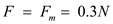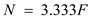…… (3)
Substitute the value of N in equation (1) and solve.…… (4)
Substitute the value of P and N in equation (2) and solve.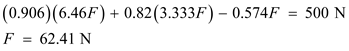Substitute the value of F in equation (4) and solve.Therefore, the smallest value of the force P required to start the block moving up the rail is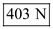.

Step: 4

(b)

Draw the free body diagram of the block when it will try to slip down.Step: 5

Take the condition of equilibrium.
Assume that the force in the positive x-direction is positive.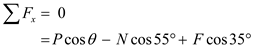Apply the equilibrium condition.…… (5)

Step: 6

Assume that the force in the positive y-direction is positive.Apply the equilibrium condition,…… (6)
The maximum value of frictional force,As the block needs to be kept from moving down, frictional force,…… (7)

Step: 7

Substitute the value of N in equation (5) and solve.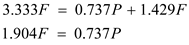…… (8)
Substitute the value of P and N in equation (6) and solve.Substitute the value of F in equation (8) and solve.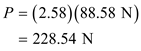Therefore, the smallest value of force P required to keep the block from moving down is.